• Call Now

1800-102-2727•

# Thermal Properties of Materials - Calorimetry, Thermal Expansion, Heat Transmission Practice Problems, FAQs

Have you ever seen that the shape of the railway tracks gets changed because of the expansion of the tracks due to increase in temperature. So to avoid this issue they often leave some gap between the two consecutive tracks so that if tracks expand it will get adjusted in that gap. This expansion of material under the application of heat is one of the thermal properties of the material. Thermal properties are exhibited by the material when heat is passed through it. In this article we will be looking at some important thermal properties.

• Heat
• Calorimetry
• Thermal Expansion
• Heat Transmission
• Practice Problems
• FAQs

## Heat

When two bodies with differing surface temperatures come into contact, heat is a type of energy that forms and travels from the body with a higher surface temperature to the one with a lower surface temperature. It is energy that is moving or in transit. Heat is not a system's property. A system does not contain heat, yet it can emit or absorb heat. It is the type of energy that controls how a body's thermal state changes and is characterised as the transfer of energy from one body to another as a result of the two bodies' different levels of heat (temperature). It transfers heat from one body's high temperature to another's low temperature.

Internal energy is the energy involved in the arrangement and haphazard movement of the atoms and molecules within a body. Heat is the portion of internal energy that is transferred from one body to another as a result of temperature differences.

Heat can be converted into other forms of energy and vice versa because it is a kind of energy.

For instance, a resistor transforms electrical energy into thermal energy, while a thermocouple does the opposite. Mechanical energy is transformed into heat energy through friction. Heat energy is transformed into mechanical energy by a heat engine.

## Calorimetry

A calorimeter is a device that is used for heat measurements, necessary for calorimetry.

The figure below shows a basic calorimeter.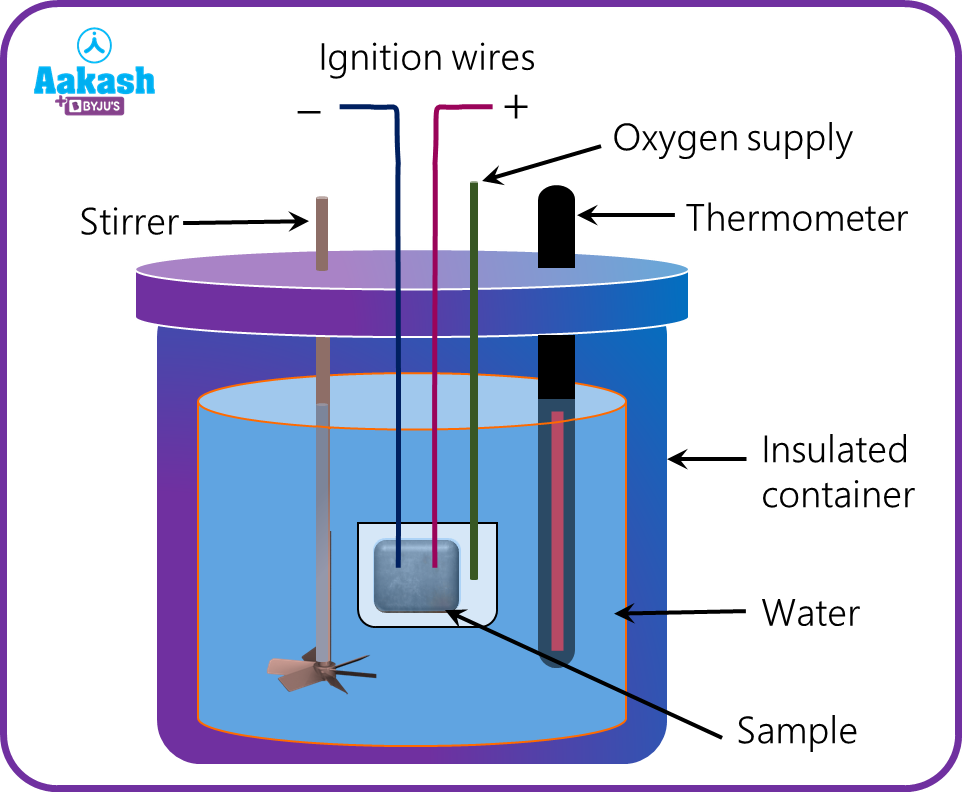It consists of an insulated chamber having ignition wires with a thermometer and a stirrer attached to it as shown. The sample placed in the vessel is burned by the aid of ignition wires and a passage through which oxygen is passed for burning. The amount of heat released by the sample is measured by measuring the temperature rise in the water and further using it along with water mass and the specific heat capacity of water. The heat loss is prevented by the insulated chamber. The stirrer ensures uniform heat distribution in the water.

Principle behind calorimetry is that in the case of an insulated system, the heat energy lost by a hot body is the same as the heat energy gained by the cold body.

The Calorimeter is based on the following formula.

We know that from the conservation of the energy at steady state situation,

Heat loss = Heat gain

q=mcΔt

Where q denotes the measure of the transferred heat into the system, m denotes the mass of substance, c denotes the specific heat capacity of the substance , Δt denotes the temperature change.

## Thermal Expansion

• Thermal expansion is the property of matter to change in shape, volume, area, and configuration in response to a change in temperature.
• It can be thought of as a photographic enlargement of the object, where the distance between any two points increases/decreases in accordance with the temperature.The graphical representation of an object at two different temperatures T and T0 (T >T0) is shown in the figure. It can be observed that the photographic enlargement is not only restricted to an increase in length, breadth, and area, but also increases the distance between any two points chosen at random.

Thermal expansion at molecular level

We can understand thermal expansion qualitatively on the molecular level. The interatomic forces in a solid can be considered as springs, as in figure below. There is an analogous relationship between spring forces and interatomic forces. Each atom vibrates about its equilibrium position. As spring is easier to stretch as compared to compress, the interatomic spring behaves in a similar manner and these are not symmetrical about equilibrium position.

With the rise in temperature, the amplitude of vibrations at atomic level increases inside the matter. This results in the increase of energies and average distances between the atoms. Therefore, the matter as a whole expands.Point to Remember

 With the rise in temperature, the amplitude of vibrations at atomic level increases inside the matter, which results in the increase of energies and average distances between the atoms. Therefore, the matter as a whole expands.

Thermal expansion in solids

Solids can expand in one, two, or three dimensions. These are known as linear expansion, areal expansion, and volume expansion, respectively.

Isotropic Solids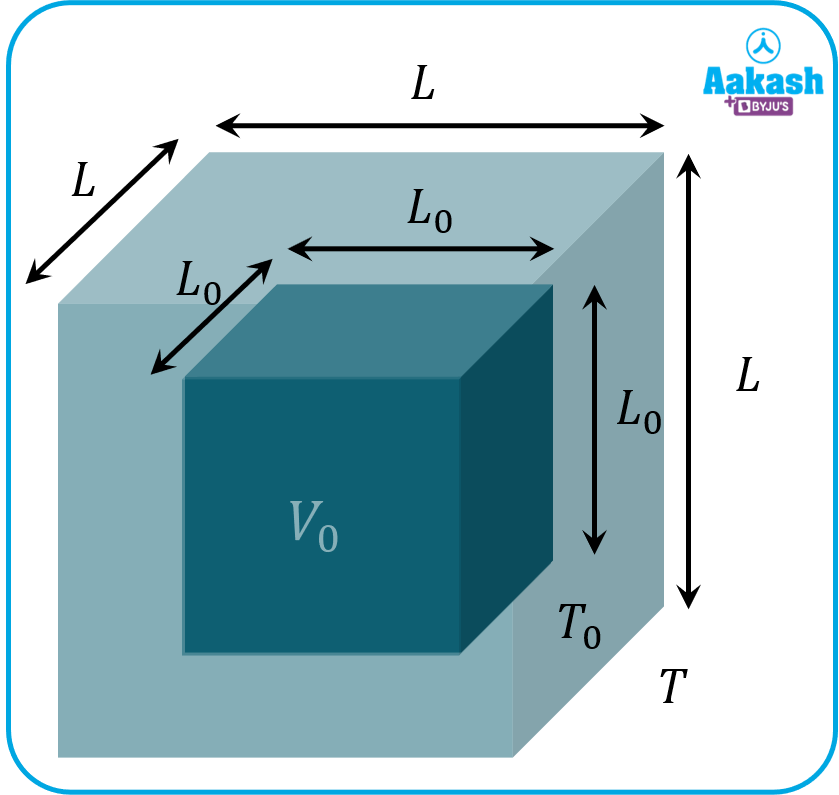The solids whose physical properties are independent of the orientation of the system are known as isotropic solids. In other words, substances that expand linearly at the same rate in every direction are known as isotropic substances.

The coefficient of linear expansion (𝛼) of an isotropic solid is the same in x, y, and z directions.

Anisotropic Solids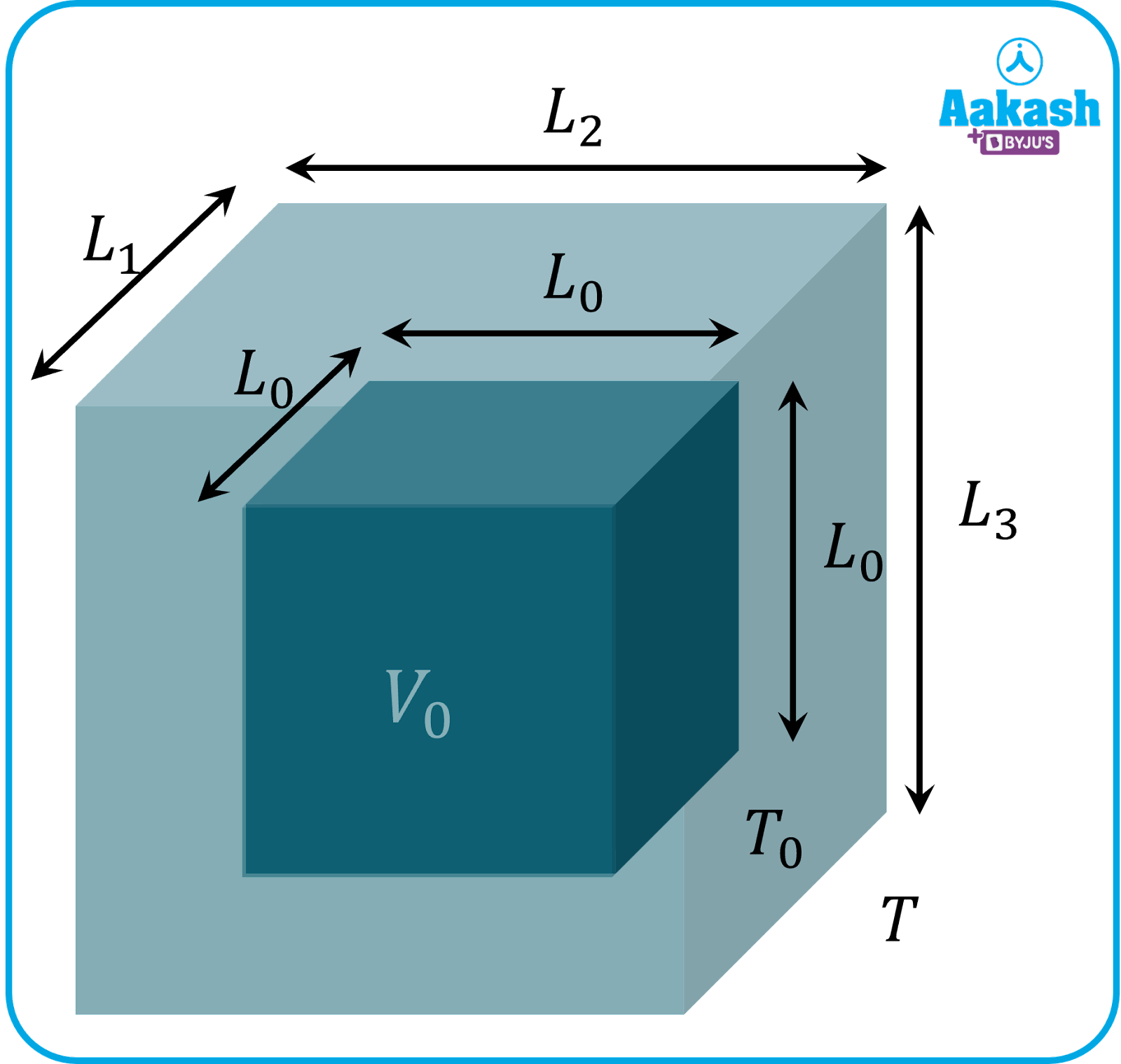The solids whose physical properties change in different orientations of the system are known as anisotropic or non-isotropic solids.

For an anisotropic solid, let 𝛼1, 𝛼2, and 𝛼3 be the coefficients of linear expansion in x, y, and z directions. Then, the coefficients of areal and volume expansion will be as follows:

𝛽 = 𝛼1 + 𝛼2

𝛾 = 𝛼1 + 𝛼2 + 𝛼3

Linear expansion

It refers to the change in the length of the body due to thermal expansion.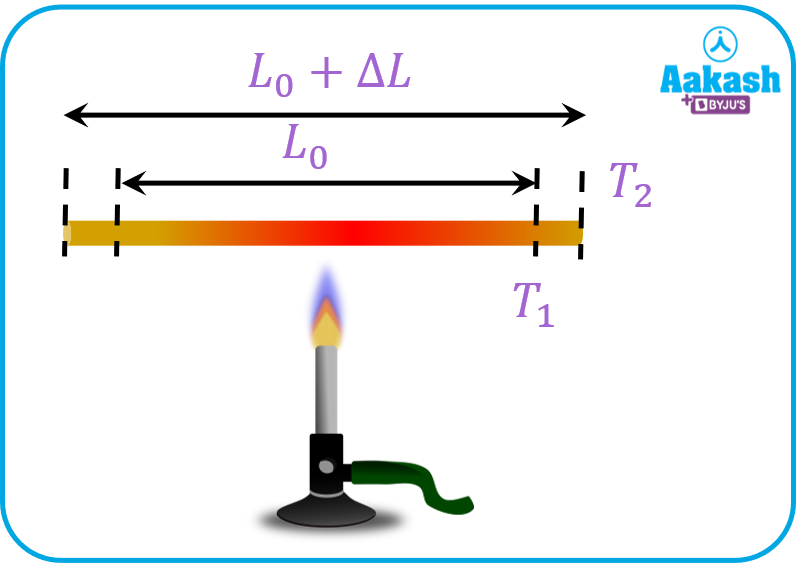Consider a rod calibrated at length L0 initially at temperature T1. Heat has been supplied to the rod for some time. The temperature of the rod rises up to T2 and its length increases by amount ΔL.

According to the principle of thermal expansion, the change in length (ΔL) depends on the following two factors.

1. LT
2. LLo

Introducing a proportionality constant,

$\mathrm{\Delta L}=\alpha {L}_{o}\mathrm{\Delta T}$

Where is coefficient of linear expansion and it is property of a material.

Units of isoC-1 or K-1

${L}_{o}+\mathrm{\Delta L}={L}_{o}+\alpha {L}_{o}\mathrm{\Delta T}$

$={L}_{o}\left(1+\alpha \Delta T\right)$

For small increments in length,

$\alpha =\frac{1}{L}\frac{dL}{dT}$

${\int }_{{L}_{o}}^{L}\frac{dL}{L}=\alpha {\int }_{{T}_{1}}^{T}dT$

$ln\left[L\right]LoL=\alpha \Delta T$

$ln\frac{L}{{L}_{o}}=\alpha \Delta T$

$⇒L={L}_{o}exp\alpha \Delta T$

Even for temperature differences in the range of 102, the value of expression αΔT is of order 10−3.

Hence, for practical purposes, one can expand the exponential series.

$L\approx {L}_{o}\left[1+\alpha \Delta T\right]$

Linear expansion coefficients for some material

 Material (10-6 / oC) Ordinary Glass 9 Aluminium 23 Brass 19 Copper 17 Iron 12 Steel 13 Tungsten 4.3 Gold 17 Quartz 0.59

Areal expansion

Areal or superficial expansion refers to the expansion in the area of an object due to the increase in temperature.

The expression for areal thermal expansion is similar to that of linear expansion.

$\mathrm{\Delta A}=\beta {A}_{o}\mathrm{\Delta T}$

= coefficient of real expansion

Ao= Initial area

A and T represents change in area and temperature respectively.

Final area A=Ao+A

$A={A}_{o}+\beta {A}_{o}\mathrm{\Delta T}$

$A={A}_{o}\left(1+\beta \Delta T\right)$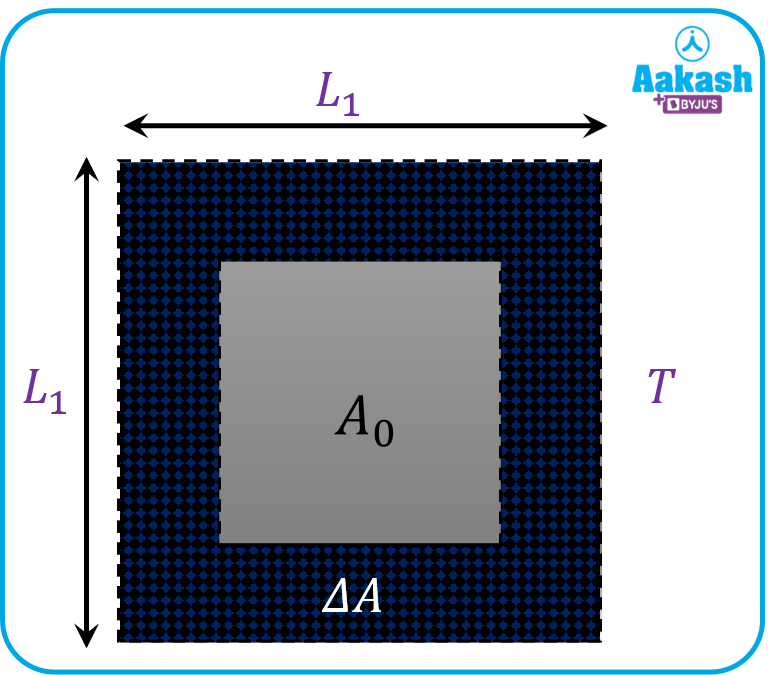Consider a case of square sheets of area A0 for simplicity. It undergoes expansion due to a rise in temperature.

L0 and L are the lengths of the sides of the sheet before and after the expansion takes place. The final value of the area of the sheet is A.

Therefore,

${A}_{o}={L}_{o}^{2}$

$A={L}^{2}={L}_{o}^{2}\left(1+\alpha \Delta T{\right)}^{2}$

$A\approx {L}_{o}^{2}\left(1+2\alpha \Delta T\right)$

$A={A}_{o}\left(1+2\alpha \Delta T\right)$

Since A=Ao(1+T)

$⇒\beta =2\alpha$

Thus the coefficient of areal expansion is twice as much as linear expansion.

Volume expansion

Volume expansion, also known as cubical expansion, is the increase in the volume of a solid due to the rise in temperature.

Similar to linear and areal expansions, the expression for volume expansion can be deduced as follows:ΔV = 𝛾VoΔT

Thus,

V = Vo+ΔV

Or

V = Vo + 𝛾VoΔT

Or

V = Vo(1 + 𝛾ΔT)

Consider a cubical solid of side L0 initially at temperature T. The temperature of this solid has been raised by an amount ΔT. It goes under volume expansion and as a result, the length of its sides increases up to L.

From the expression of linear expansion, we can write it as:

${L}^{3}={L}_{o}^{3}\left(1+\alpha \Delta T{\right)}^{3}$

$⇒V={V}_{o}\left(1+\alpha \Delta T{\right)}^{3}$

Using binomial expansion as T<<1,

$V={V}_{o}\left(1+3\alpha \Delta T\right)$

$⇒\gamma =3\alpha$

Thus the coefficient of volume expansion is thrice as much as linear expansion.

It can be concluded that for solids,

$\alpha :\beta :\gamma =1:2:3$

## Heat Transmission

There are three major modes of heat transfer:

1. Conduction:
1. Heat conduction is the flow of internal energy from a region of higher temperature to that of a lower temperature by the interaction of adjacent particles (atoms, molecules, ions, electrons, etc.) in the intervening space.
2. Heat transfer happens only due to the collisions between the neighbouring molecules.
3. The rate of heat transfer through a medium via conduction is given by Fourier’s Law of Heat Conduction,

$Q=-kA\frac{dT}{dx}$

Where k = thermal conductivity of the material

A = cross-sectional area

dTdx= temperature gradient across the medium.

1. Convection:
1. Heat transfer by the bulk movement of molecules within fluids such as liquids and gases.
2. Convection heat transfer is further of two types i.e. natural and forced convection depending upon the involvement of external factors.
3. The rate of heat transfer by convection is given by,

$q=hA\left(T-{T}_{o}\right)$

h = Convection coefficient

A = Surface area of the body

T = Temperature of the body

T0 = Temperature of the surrounding

1. It is a type of heat transfer that does not require any intervening medium.
2. Radiation heat transfer uses electromagnetic waves to transfer heat from one body to another.
3. There are many laws that govern radiation heat transfer but the most important is Stefan Boltzmann law.
4. According to Stefan Boltzmann law, radiant energy emitted by a body is given by

E = ε𝜎AT4

Where ε= emissivity of the material.

A= surface area of the body.

𝜎= Stefan’s constant = 5.67 × 10-8 W m-2K-4

T= absolute temperature of the body

## Practice Problems

Q. 1 g ice is mixed with 1 g of steam. After thermal equilibrium is reached, find the temperature of the mixture.

(Latent heat of fusion of ice; Lice=80 cal/g, latent heat of condensation of vapour; Lv=540 cal/g, Specific heat capacity of water; c=1 cal/g oC)

A.

We know,

Latent heat of fusion of ice; Lice=80 cal/g

Latent heat of condensation of vapour; Lv=540 cal/g

Specific heat capacity of water; c=1 cal/g oC

Heat supplied by steam if it is to condense totally and convert into water at 100 oC.

Heat required by ice to convert totally into water at 0 oC

${Q}_{2}=1×{L}_{ice}$

Heat required by ice to convert totally into water at 100 oC

=80+100

=180 cal

Since Q1>Q3, entire steam will not condense and the final temperature of mixture of water and steam will be 100 oC.

Q. If the temperature of an iron rod of length 1 m changes by 100oC, what will be the percentage change in its length?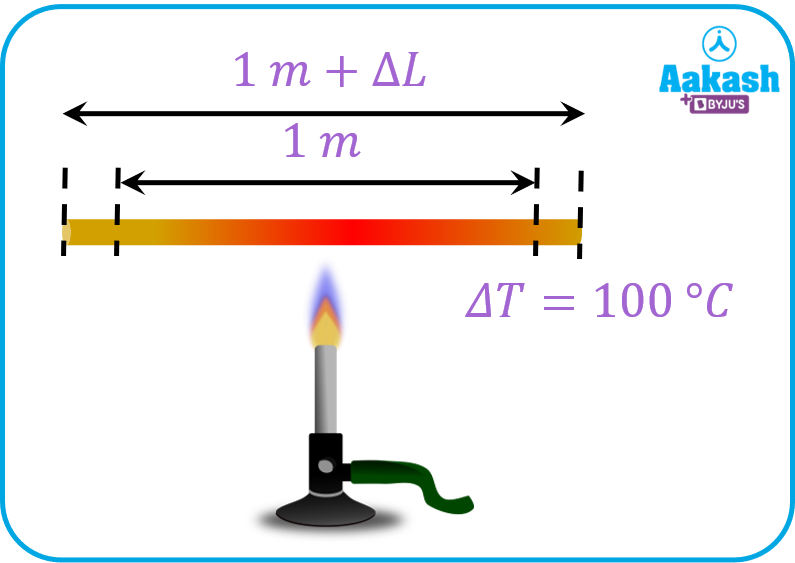A.

Given,

Percentage change in length $=\left(\frac{\mathrm{\Delta L}}{L}×100\right)%$

$=\left(\frac{{\alpha }_{iron}{L}_{o}\mathrm{\Delta T}}{{L}_{o}}×100\right)%$

$=\left(12×{10}^{-5}×{10}^{2}×100\right)%=1.2%$

Q. Find the ratio of the lengths of an iron rod and an aluminium rod for which the difference in the lengths is independent of temperature.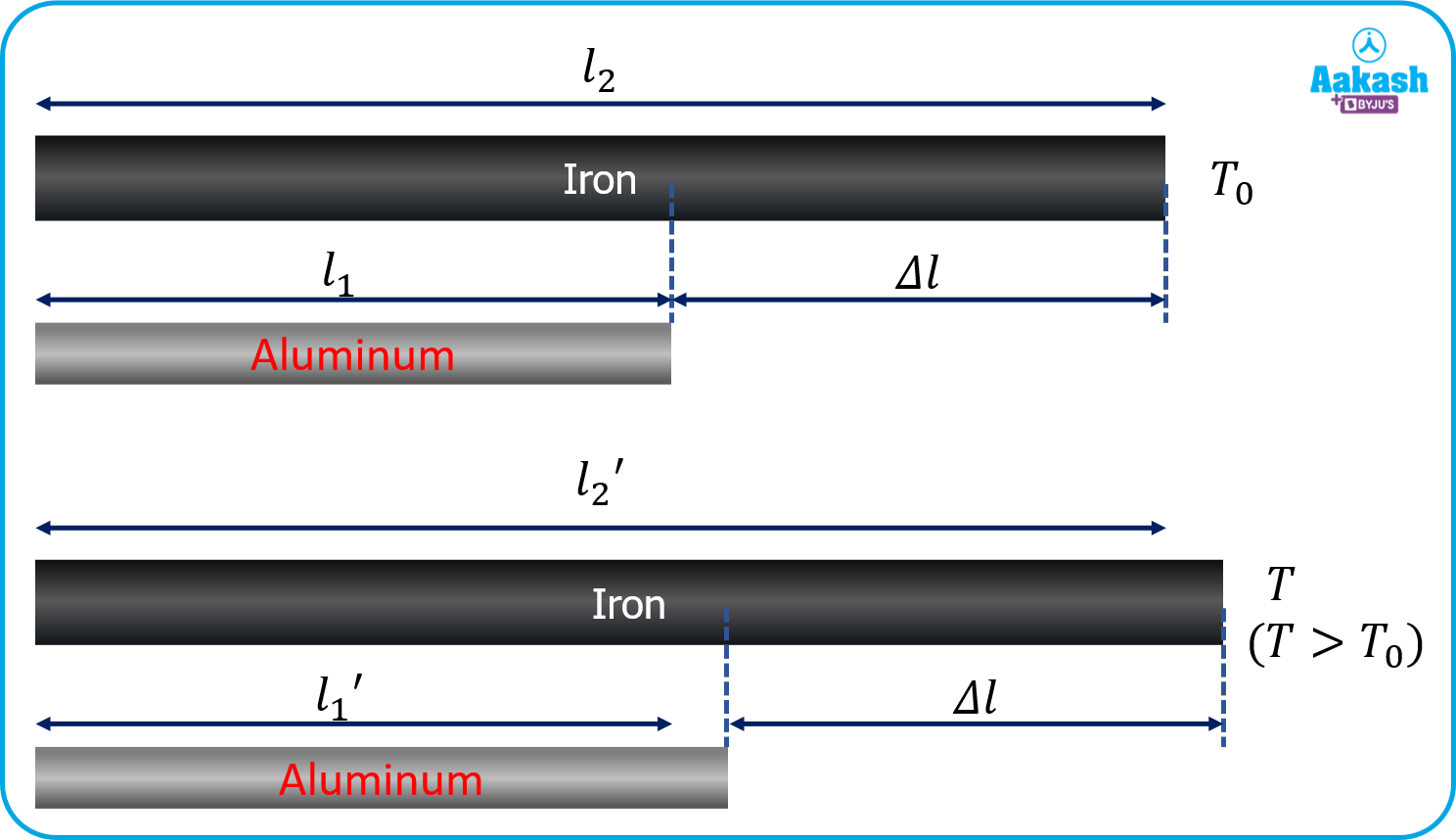A.

Temperatures of both the rods were raised from T0 to T, which results in the increment of their lengths.

Assuming that the lengths of aluminium and iron rods at temperature T0 are l1 and l2, respectively. In response to the rise in temperature, their lengths are increased to l1’ and l2’, respectively.

It is also given that the difference in the lengths of both the rods (l1 − l2) is independent of temperature.

Therefore,

${l}_{1}-{l}_{2}={l}_{1}\text{'}-{l}_{2}\text{'}$

$⇒{l}_{1}-{l}_{1}\text{'}={l}_{2}-{l}_{2}\text{'}$

$⇒\mathrm{\Delta }{l}_{1}=\mathrm{\Delta }{l}_{2}$

${\alpha }_{Al}{l}_{1}\mathrm{\Delta T}={\alpha }_{Fe}{l}_{2}\mathrm{\Delta T}$

$⇒{\alpha }_{Al}{l}_{1}={\alpha }_{Fe}{l}_{2}$ [Since T=T-To is same for both the rods]

$\frac{{l}_{2}}{{l}_{1}}=\frac{{\alpha }_{Al}}{{\alpha }_{Fe}}=\frac{23×{10}^{-5}}{12×{10}^{-5}}=\frac{23}{12}$

Hence, the ratio of the lengths of iron and aluminium rods is 23:12

Q. A thin brass sheet which is at a temperature of 10oC have same surface area as that of a thin steel sheet at 20oC. Find the common temperature at which both would have the same area.

Coefficient of areal expansion for brass and steel are 1010-6/oC and 2010-6/oC respectively.

A. We know ,

Final area A=Ao(1+T)

where Ao is the initial area.

Given:

Ao is the same for both brass and steel sheet at 10oC and 20oC respectively.

Let at temperature T, both have the same area A.

${A}_{o}\left(1+{\beta }_{b}\mathrm{\Delta }{T}_{1}\right)={A}_{o}\left(1+{\beta }_{s}\mathrm{\Delta }{T}_{2}\right)$

${A}_{o}\left(1+{\beta }_{b}\left(T-10\right)\right)={A}_{o}\left(1+{\beta }_{s}\left(T-20\right)\right)$

$⇒\frac{{\beta }_{b}}{{\beta }_{s}}=\frac{T-20}{T-10}$

$⇒\frac{10×{10}^{-6}}{20×{10}^{-6}}=\frac{T-20}{T-10}$

$⇒T={30}^{o}C$

## FAQs

Q. Why are metals used in calorimeters?
A.
Metals are usually good conductors of heat and have low specific heat. Thus they reach equilibrium temperature quickly.

Q. Can a process occur without any heat transfer across the system?
A.
Yes. Adiabatic process occurs without any heat transfer across the system.

Q. Is it possible that the temperature of a system decreases with the addition of heat?
A.
Yes, it is possible that the temperature of the system may decrease upon heat addition. This occurs in polytropic processes which have negative specific heat. Basically the system does more work than the amount of heat added and due to this the internal energy of the system decreases, thus resulting in decrease in temperature of the system.

Q. Two rods having the same material and initial temperature but different lengths are heated to the same temperature. For which rod change in length will be greater?
A.
Change in length will be more for longer rod as compared to shorter rod having same material and same initial temperature.Talk to our expert
Resend OTP Timer =
By submitting up, I agree to receive all the Whatsapp communication on my registered number and Aakash terms and conditions and privacy policy